# Set Partition Problems

This is my first post of 2019 and my first post in a while. There was one posted a few months ago, but not really geared towards the algorithms and learning focus of the site. I have been doing a lot of coding in my spare time, but honestly life has just gotten in the way. Its not a bad thing, but life is life and sometimes I have to prioritize the things. In particular, I have been having ideas and actually coding things up but the time it takes to clean up code, write a blog entry and finding a nice way to visualize these things has been something that I haven’t been able to really focus on as much as I’ve wanted to.

That said, I want to talk to you about the Set Partition Problem today. You can go to Wikipedia to get more information about this problem, but I will give you a brief introduction to it and then talk about two different approaches to it. The problem assumes that we are given as input a (multi)-set S. The reason we say it is a multi-set and not a simple set is because we can have the same element appear multiple times in the set. So if S1 = {2} and S2 = {2, 2}, then although as sets they are both equal to the set {2} = S1, as multi-sets allow for multiple instances of an element. The elements of S are assumed to be positive integers.

So given this multi-set S, we ask the question of can the elements of S be divided into two smaller multi-sets, C1 and C2 where

• C1 [union] C2 = S
• C1 [intersect] C2 = [empty set]
• [Sigma]_[x in C1] = [Sigma]_[x in C2].C1 [union] C2 = S, C1 [intersect] C2 = [empty set], and [Sigma]_[x in C1] = [Sigma]_[x in C2]

The first two bullets above say that the sets C1 and C2 form a partition of S. The third bullet says that the sums of the elements in the two children multi-sets are equal.

This problem is known to be NP Complete. This means that it is one of the more difficult decision problems. Because of this finding an algorithm that solves this problem exactly will generally take a long running time. And finding an algorithm that runs quickly will more than likely be incorrect in some instances.

I will show you two approaches to this problem. One is based on Dynamic Programming (DP) and one is based on Greedy Algorithms. The DP version solves the problem exactly but has a slow running time. The greedy algorithm is fast but is not guaranteed to always give the correct answer.

Dynamic Programming is based on the principle of optimality. This says that in order to have a correct solution to the overall problem, we need optimal solutions to all subproblems of this problem. This is done by keeping track of a table which can be used for looking up these subproblems and the optimal solutions to these subproblems.

For this problem, we will build a table where the rows represent the final sums and columns represent subsets of the given multi-set (containing the first 0…n elements). The question we are repeatedly asking is “can we find a subset of the set in this column whose sum is exactly the given rowsum?” Here is an example of the DP algorithm on the multi-set {5, 6, 5, 6, 7}.

Greedy Algorithms are generally based on sorting the elements based on some principle and using that to try to answer the underlying question. The main problem with this approach is that it is very short sided because they do not look at the overall picture. Such algorithms are known for finding local optima that are not always globally optimal. The benefit to these problems though is that they are generally easier to code and easier to understand.

For the Set Partition Problem , the Greedy approach is to sort elements in descending order. Once this is done, the goal is to keep two subsets while iterating through the array, adding the element to the smaller of the two sets whenever possible.

For more examples, check out Set Partition Problems

# Unidirectional TSP Puzzles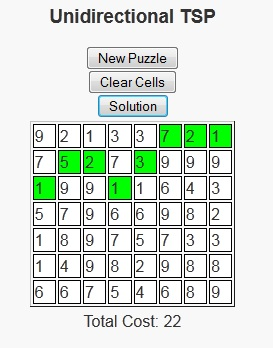As we’ve entered the late spring into early summer season, I’ve found myself wanting to go out more to sit and enjoy the weather. One of these days recently I sat in the park with a good book. On this occurrence, I decided not to go with a novel as I had just finished “Incarceron“, “The Archer’s Tale“, and “14 Stones” – all of which were good reads, but I felt like taking a break from the novels.

Just as a side note, 14 Stones is a free book available on smashwords.com and I’ve now read about 6 books from smashwords.com and haven’t been disappointed yet. My favorite is still probably “The Hero’s Chamber” because of the imagery of the book, but there are some well written ebooks available there by some good up and coming writers for a reasonable price, with some being free.

So with the desire to read, but not being in the mood for novels I decided to pick up one of my non-text but still educational books that make me think. This day it was “Programming Challenges“. I browsed through the book until I found one that I could lay back, look at the water, and think about how to solve it.

The programming puzzle the peaked my interest was called “Unidirectional TSP”. We are given a grid with m rows and n columns, with each cell showing the cost of using that cell. The user is allowed to begin in any cell in the first column and is asked to reach any cell in the last column using some minimum cost path. There is an additional constraint that once a cell is selected in a column, a cell in the next column can only be chosen from the row directly above, the same row, or the row directly below. There is a javascript version of this puzzle available here.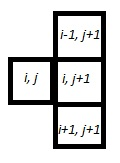Fundamentally, the problem is asking for a path of shortest length. Many shortest length problems have a greedy structure, but this one gained my interest because the greedy solution is not always optimal in this case. So I took a moment to figure out the strategy behind these problems. Once I had that solution, I decided that it would be a good program to write up as a puzzle.

In this puzzle version, users will click the cells they wish to travel in each column in which case they will turn green (clicking again will turn them white again). Once the user clicks on a cell in the last column, they will be notified of whether or not they have chosen the minimum path. Or if users are unable to solve a puzzle, the “Solution” button can be pressed to show the optimal path and its cost.

# Triangle Sum Puzzle

This is probably a consequence of being a mathematician, but I have always enjoyed number puzzles. I think that there is a general simplicity and universality in numbers that are not present in things like word puzzles, where the ability to reach a solution can be limited to the vocabulary of the user.

The fact that these are puzzles and not simply homework exercises also helps because we often find people sharing difficulties and successes stories over the water cooler or at the lunch table. The fact that many of these math puzzles can teach some of the same concepts as homework problems (in a more fun and inclusive way) is generally lost on the user as their primary interest is generally on solving the puzzle in front of them, or sometimes solving the more general form of the puzzle.

Today’s post is about a puzzle that was originally shared with me over a lunch table by a friend who thought it was an interesting problem and asked what I thought about it. I didn’t give the puzzle much further thought (he had correctly solved the puzzle) until I saw it again in “Algorithmic Puzzles” by Anany Levitin and Maria Levitin. It was then that I thought about the more general form of the puzzle, derived a solution for the problem, and decided to code it up as a script for my site.

Below is a link to the puzzle: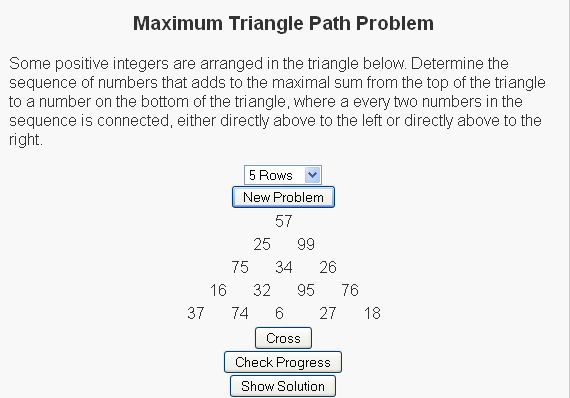We have a set of random numbers arranged in a triangle and the question is to find the path of maximum sum from the root node (the top node) to the base (one of the nodes on the bottom row) with the rules that
(1) Exactly one number must be selected from each row
(2) A number can only be selected from a row if (a) it is the root node or (b) one of the two nodes above it has been selected.

For the sample
So for the sample problem in the picture, the maximal path would go through nodes 57, 99, 34, 95, and 27.

For more of these puzzles check out the script I write here and be sure to let me know what you think.

# The Bridge Crossing Problem

Most puzzles are fun in their own right. Some puzzles are so fun that they have the added benefit that they are likely to come up in unexpected places, like maybe in a job interview. I was recently reading a paper by Günter Rote entitled “Crossing the Bridge at Night” where Rote analyzes such a puzzle. Upon finishing the paper, I decided to write a script so that users could see the general form of this puzzle.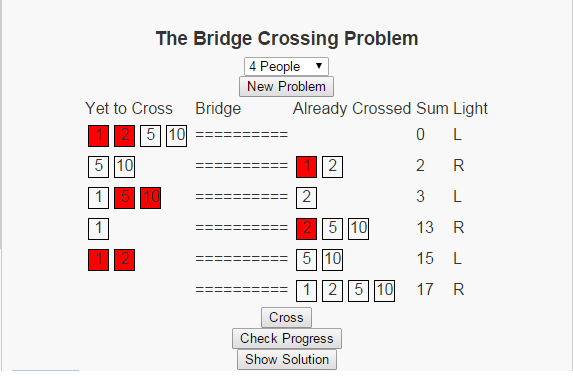The problem can be stated as follows: There is a set of people, lets make the set finite by saying that there are exactly n people, who wish to cross a bridge at night. There are a few restrictions that make crossing this bridge somewhat complicated.

• Each person has a travel time across the bridge.
• No more than two people can cross the bridge at one time.
• If two people are on the bridge together, they must travel at the pace of the slower person.
• There is only one flashlight and no party (of one or two people) can travel across the bridge without the flashlight.
• The flashlight cannot be thrown across the bridge, and nobody can go to the store to purchase another flashlight

The image above shows the optimal solution when the 4 people have travel times of 1, 2, 5, and 10. The script I have written allows users to work with different numbers of people with random travel times. Give it a try and see if you can spot the patterns in the solution.

# The A* Algorithm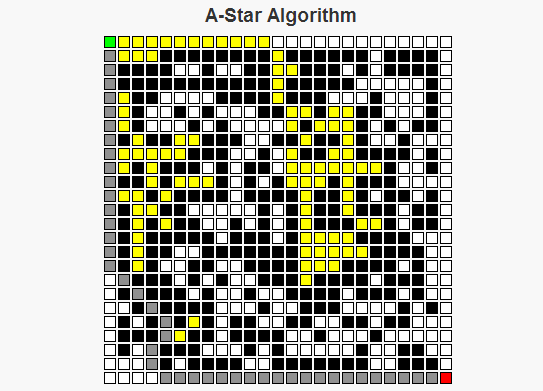As a child I remember traveling on road trips, sitting in the back of a car trying to do my best to keep myself busy for what would occupy the next six to ten hours of my life. One of the things I grew to like were the simple maze books that were sold on the magazine racks at some of the gas stations where we’d stop for food. There are two basic strategies I employed for solving these mazes: For simpler mazes, I could generally just take a look at the overall maze structure, decide upon a path through the maze, then write a path without any mistakes. For more complex mazes though, I would generally begin a route that looks the most promising. If that route reaches a point where I can see that it will be impossible to finish, then I’d go back to where the decision was made, exclude the route I had just tried, and select the “most promising” remaining route. I’d continue this process until I had completed the maze, or until it became simple enough for me to solve the maze using only my memory.

The A* Algorithm works in a similar manner to the second approach I just described. We begin at a starting point, and consider where to move next from that starting point. The set of possible options for this next move is determined by the neighbor function for a given cell. For each neighbor the algorithm estimates the length of the route through that cell by calculating the sum of two values, f(n) = g(n) + h(n), where

g(n) is the (known) cost to travel from the starting point to the cell n.
h(n) is the (approximate) cost to travel from the cell n to the final cell.

The sum g(n) + h(n) allows us to approximate the total cost of a route through the cell n.

The A* algorithm begins at the starting position of the maze. There are two sets we will be considering throughout the process of determining the optimal route, called the closedSet and the openSet. The elements of closedSet are the nodes whose total distance from the starting position has been calculated, whereas the elements of openSet represents nodes whose total distance is still under consideration. There is also a map called prev which is used to reconstruct the path. Below is how the algorithm operates:

A* Algorithm Pseudocode
closedSet is the empty set
openSet = {start}
prev is the empty set

While there are still elements in openSet,
Find the element c* in openSet with the lowest value f(c).
If c* is the target position
Reconstruct the path.
Else If c* is not the target position,
Remove c* from openSet
If n is not in openSet, or if n is in openSet and temp_g(n) < g(n),                     Set prev(n) = c*                     Set g(n) = temp_g(n)                     Set f(n) = g(n) + h(n).                     if n is not in openSet                          add n to openSet.      End If End While End AlgorithmAn important question becomes what makes a good heuristic function to approximate the distance to the goal. This can lead to an in depth discussion based on the word "good", but the necessary condition for any heuristic is that it NEVER over-estimates the cost of the path from the cell to the goal. Some examples of possible heuristics for mazes are the Euclidean Distance (the square root of the sum of the squares of the horizontal and vertical differences in distances, i.e. dE(x, y) =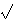(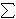i(xi – yi)2) and TaxiCab Distance (the sum of the differences in the horizontal and vertical dimensions, i.e. dT(x, y) =i|xi – yi|). Both of these are feasible metrics for heuristics on a maze. Other herusitics, like h(n) = 0 for all n are possible, but doing this would make the algorithm treat all cells equally and ignore the heuristic part of the A* algorithm, turning it into Dijkstra’s algorithm.